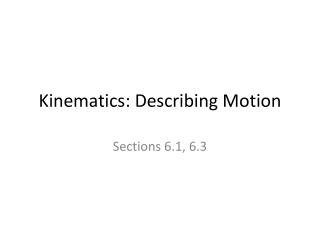DownloadDownload PresentationKinematics: Describing Motion

Kinematics: Describing Motion

Download PresentationKinematics: Describing Motion

- - - - - - - - - - - - - - - - - - - - - - - - - - - E N D - - - - - - - - - - - - - - - - - - - - - - - - - - -
Presentation Transcript

1. Kinematics: Describing Motion Sections 6.1, 6.3

2. Reminders • Lab this week: LAB A3-FF: Free Fall • Mallard-based reading quiz due prior to class on Thursday • I left extra credit items in my office; come get them after class if you wish.

3. Uniformly Accelerated Motion • Interpreting graphs • Equations: • vinst = vo + at • x = xo+ vot + ½ at2 • vinst2– vo2 = 2aΔx • Vectors • Sign conventions • Sample problem…

4. Graph Review 1 Which of the following statements most accurately describes the motion of bicycle A during the first 5 seconds? • a. Bicycle A is constantly at rest. • b. Bicycle A is moving with a constant speed • c. Bicycle A is traveling up hill • d. Bicycle A is moving in a direction opposite that of bicycle B

5. Graph Review 2 Which of the following statements most accurately describes the motion of bicycle B in comparison to bicycle A at t=5s? • a. Bicycle B is going faster than bicycle A. • b. Bicycle A is passing bicycle B. • c. Bicycle B is speeding up. • d. Bicycle B is moving in a direction opposite that of bicycle A.

6. Graph Review 3 • In a position-time graph (position in cm on the y-axis and time in s on the x-axis) of a constant-motion toy car the y-intercept is -3. What is/are the unit(s) on the -3? • a. cm • b. cm/s • c. cm/s2 • d. s

7. Graph Review 4 • In a position-time graph (position in cm on the y-axis and time in s on the x-axis) of a constant-motion toy car the y-intercept is -3. What is the physical interpretation of -3 along with its units? • a. the car’s position at t=3s • b. the car’s position at t=0s • c. the car’s position 3s before the clock starts • d. none of these

8. Equations of Motion Constant Motion (a = 0) Uniformly Accelerated Motion vinst = vo + at x = xo + vot + (½)at2 vinst2 – vo2= 2aΔx • x = xo + vavet General steps for solving simple kinematic (motion) problems: Determine type of motion – constant or uniformly accelerated motion. Identify that for which you are looking. Identify the information you have. Find an appropriate relationship between all the variables in problem. Solve the relationship for the unknown variable. Insert quantities (including units) and solve (including units). Check to see if your solution seems reasonable.

9. Graphs of Motion Constant Motion Uniformly Accelerated Motion P-T graphs Curved Tangent to curve = velocity Area under curve meaningless V-T graphs Non-horizontal lines Slope = acceleration Slope does not equal 0 Area under the line = displacement Do not give position • P-T graphs • Linear • Slope = velocity • Area under line meaningless • V-T graphs • Horizontal lines • Slope = acceleration • Slope = 0 • Area under line = displacement • Do not give position

10. Equation Review 1 A rock at rest is dropped from a bridge and falls 11.2m before reaching the water.The magnitude of the acceleration due to gravity is 9.81m/s2. How long does it take the rock to reach the water below? Ignore the presence of wind resistance. • a. 1.14s • b. 1.51s • c. 2.28s • d. none of these

11. Equation Review 2 How fast is the rock in the last question going when it hits the water? • a. 11.2m/s • b. 14.8m/s • c. 22.4m/s • d. none of these answers

12. Position, Distance, Displacement • Position – magnitude and direction (vector) from some arbitrary location. • Distance • A scalar quantity • Measured along path traveled • Displacement – measures change in position. • A vector quantity • The straight-line distance between where one starts motion and where one ends motion.

13. Velocity and Speed • Speed = |velocity| • Instantaneous velocity versus average velocity • Tangents to curved P-T graphs are instantaneous velocity. • vinst= vo + at • Average velocity (caution!examplesnext time) • vave = (change in displacement)/(time)